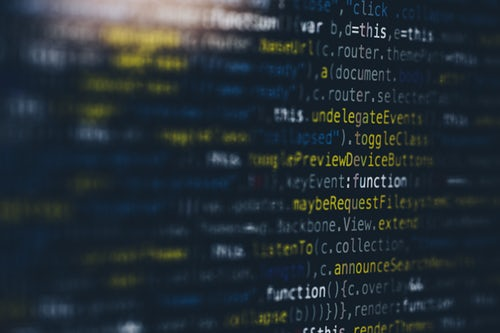# TensorFlow for Deep Learning (Part 1)

· AI Zone · Tutorial
Save
12.21K Views

This article was first published on the Knoldus blog.TensorFlow is a free and open-source software library for dataflow and differentiable programming across a range of tasks. It is a symbolic math library and is also used for machine learning applications such as neural networks. It is used for both research and production at Google. TensorFlow was developed by the Google Brain team for internal Google use.

Deep learning is a particular kind of machine learning that achieves great power and flexibility by learning to represent the world as a nested hierarchy of concepts, with each concept defined in relation to simpler concepts, and more abstract representations computed in terms of less abstract ones.

## How Does Deep Learning Work?

A deep learning model is designed to continually analyze data with a logic structure similar to how a human would draw conclusions. To achieve this, deep learning uses a layered structure of algorithms called an artificial neural network (ANN). The design of an ANN is inspired by the biological neural network of the human brain. This makes for machine intelligence that's far more capable than that of standard machine learning Models. It's a tricky prospect to ensure that a deep learning model doesn't draw incorrect conclusions (which is probably what keeps Elon up at night), but when it works as it's intended to, functional deep learning is a scientific marvel and the potential backbone of true artificial intelligence.

• Machine learning uses algorithms to parse data, learn from that data, and make informed decisions based on what it has learned
• Deep learning structures algorithms in layers to create an “artificial neural network” that can learn and make intelligent decisions on its own
• Deep learning is a subfield of machine learning. While both fall under the broad category of artificial intelligence, deep learning is what powers the most human-like artificial intelligence

Before writing actual deep learning applications, we have to understand below concepts from TensorFlow as

1. Constant
2. Variable
3. Placeholder
4. Loss Calculation
5. Loss Reduction

## Constants

Constant can be created using tf.constant() function.

``tf.constant( value,dtype=None, shape=None, name='Const', verify_shape=False )``
Args:
• value: A constant value (or list) of output type dtype.
• dtype: The type of the elements of the resulting tensor.
• shape: Optional dimensions of resulting tensor.
• name: Optional name for the tensor.
• verify_shape: Boolean that enables verification of a shape of values.
``````import tensorflow as tf
#Build computational graph
node1 = tf.constant(3.0,tf.float32)
node2 = tf.constant(4.0,tf.float32)
output = node1 + node2
#Run computational graph
sobj = tf.compat.v1.Session()
sobj.run(output)``````

Session: A session is basically the backbone of a TensorFlow program. A session fires up the program to get the constants ready and perform the desired operation. It can be done in the following way.

## PlaceHolder

A placeholder is simply a variable that we will assign data to at a later date. It allows us to create our operations and build our computation graph, without needing the data. In TensorFlow terminology, we then feed data into the graph through these placeholders.

``````tf.placeholder(
dtype,
shape=None,
name=None
)``````
Args:
• dtype: The type of elements in the tensor to be fed.
• shape: The shape of the tensor to be fed (optional). If the shape is not specified, you can feed a tensor of any shape.
• name: A name for the operation (optional).
``````import tensorflow as tf
#Build computational grapg
node1 = tf.compat.v1.placeholder(tf.float32)
node2 = tf.compat.v1.placeholder(tf.float32)
output = node1 + node2
#Run computational graph
sobj = tf.compat.v1.Session()
sobj.run(output,{node1:[1,3],node2:[4,5]})``````

## Variable to Calculate the Loss

A TensorFlow variable is the best way to represent shared, persistent state manipulated by your program. A variable maintains state in the graph across calls to run(). You add a variable to the graph by constructing an instance of the class Variable

``````import tensorflow as tf

#Model Parameter
w = tf.compat.v1.Variable([.3],tf.float32)
b = tf.compat.v1.Variable([-.3],tf.float32)

#Input and Output
x = tf.compat.v1.placeholder(tf.float32)

linear_model = w * x + b

y = tf.compat.v1.placeholder(tf.float32)

#loss function

squared_delta = tf.square(linear_model-y)
loss = tf.reduce_sum(squared_delta)

init = tf.global_variables_initializer()

#Run computational graph
sobj = tf.compat.v1.Session()
sobj.run(init)

sobj.run(loss,{x:[1,2,3,4],y:[0,-1,-2,-3]})``````

## Loss Reduction

We use a loss function to determine how far the predicted values deviate from the actual values in the training data. We change the model weights to make the loss minimum, and that is what training is all about. Gradient Descent is a learning algorithm that attempts to minimize some error

TensorFlow has a whole set of types of optimization and has the ability for you to define your own as well

• MomentumOptimizer
• FtrlOptimizer
• RMSPropOptimizer
``````import tensorflow as tf

#Model Parameter
w = tf.compat.v1.Variable([.3],tf.float32)
b = tf.compat.v1.Variable([-.3],tf.float32)

#Input and Output
x = tf.compat.v1.placeholder(tf.float32)

linear_model = w * x + b

y = tf.compat.v1.placeholder(tf.float32)

#loss function

squared_delta = tf.square(linear_model-y)
loss = tf.reduce_sum(squared_delta)

#optimiser

train = optimiser.minimize(loss)

init = tf.global_variables_initializer()

#Run computationalgraph
sobj = tf.compat.v1.Session()
sobj.run(init)

for i in range(1000):
sobj.run(train,{x:[1,2,3,4],y:[0,-1,-2,-3]})

sobj.run([w,b])``````